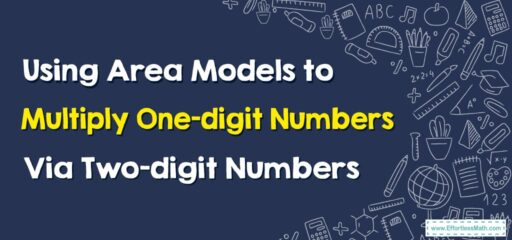# How to Use Area Models to Multiply One-Digit Numbers By Two-digit Numbers

Using area models to multiply one-digit numbers by two-digit numbers is a great way to visualize the multiplication process.## A Step-by-step Guide to Using Area Models to Multiply One-Digit Numbers By Two-digit Numbers

Using area models to multiply one-digit numbers by two-digit numbers can help students visualize the multiplication process. Here’s a step-by-step guide using the example $$5 x 12$$.

### Step 1: Draw the Area Model

Start by drawing a large rectangle. This rectangle will represent the entire multiplication problem.

### Step 2: Divide the Rectangle

Next, divide the rectangle into two parts. This is because we’re multiplying by a two-digit number (12). The larger part represents the tens place (10), and the smaller part represents the ones place (2). Label each section.

It would look something like this:

-----------------
|     10     |  2  |
-----------------


### Step 3: Write the One-Digit Number

On the side of the rectangle, write the one-digit number you’re multiplying (5).

     -----------------
5  |     10     |  2  |
-----------------


### Step 4: Calculate the Area

The area of a rectangle is found by multiplying its length by its width. So, multiply the one-digit number (5) by each part of the two-digit number (10 and 2).

For the first part of the rectangle: $$5 x 10 = 50$$
For the second part of the rectangle: $$5 x 2 = 10$$

Write these products inside the respective parts of the rectangle.

     -----------------
5  |     50     |  10  |
-----------------


### Step 5: Add the Areas

Finally, add the two areas together. In this case, $$50 + 10 = 60$$.
So, $$5 x 12 = 60$$.
Area models are a great way for students to visualize multiplication, breaking down complex problems into simpler, more manageable parts. It’s especially useful for students who are visual learners.

### What people say about "How to Use Area Models to Multiply One-Digit Numbers By Two-digit Numbers - Effortless Math: We Help Students Learn to LOVE Mathematics"?

No one replied yet.

X
30% OFF

Limited time only!

Save Over 30%

SAVE $5 It was$16.99 now it is \$11.99